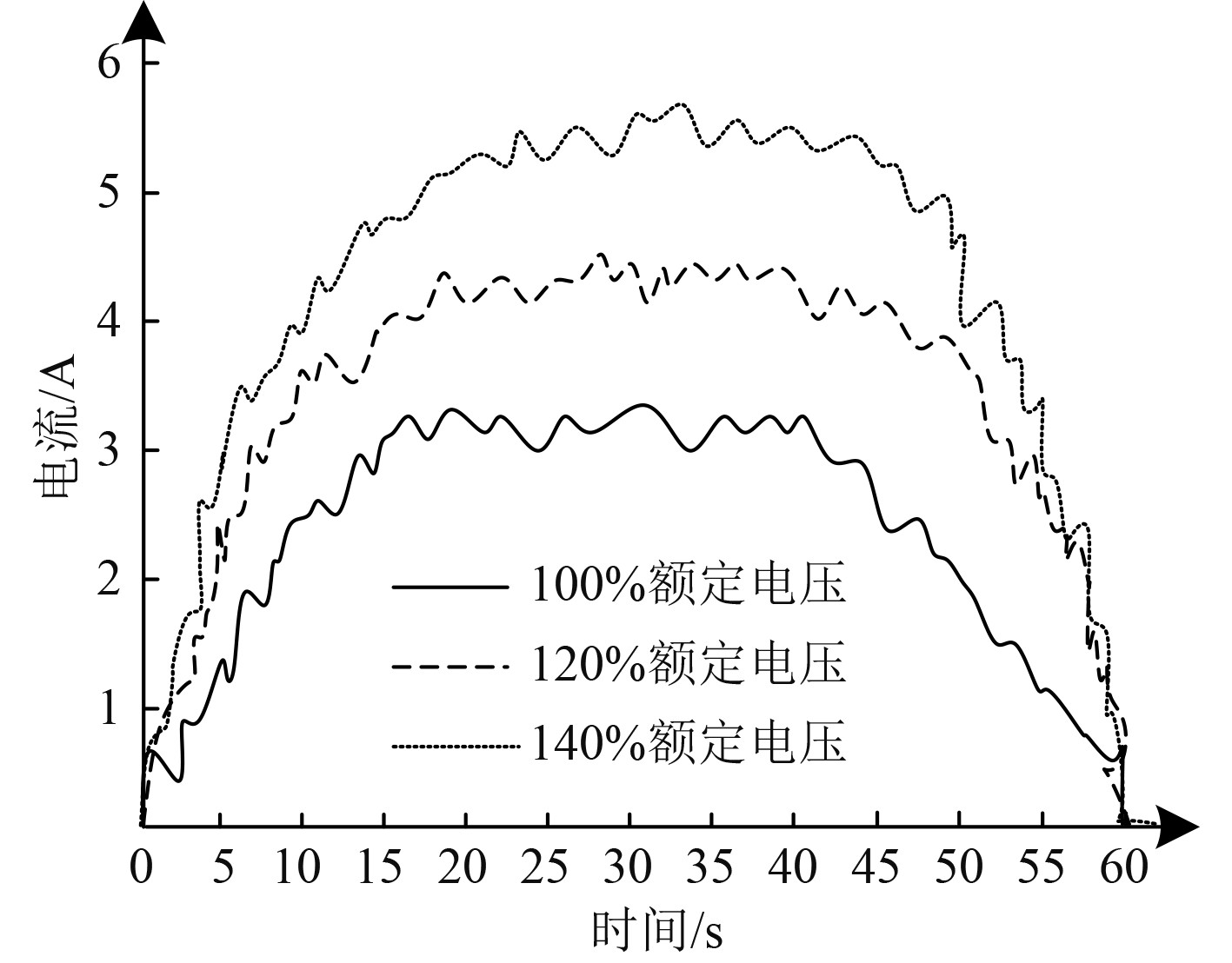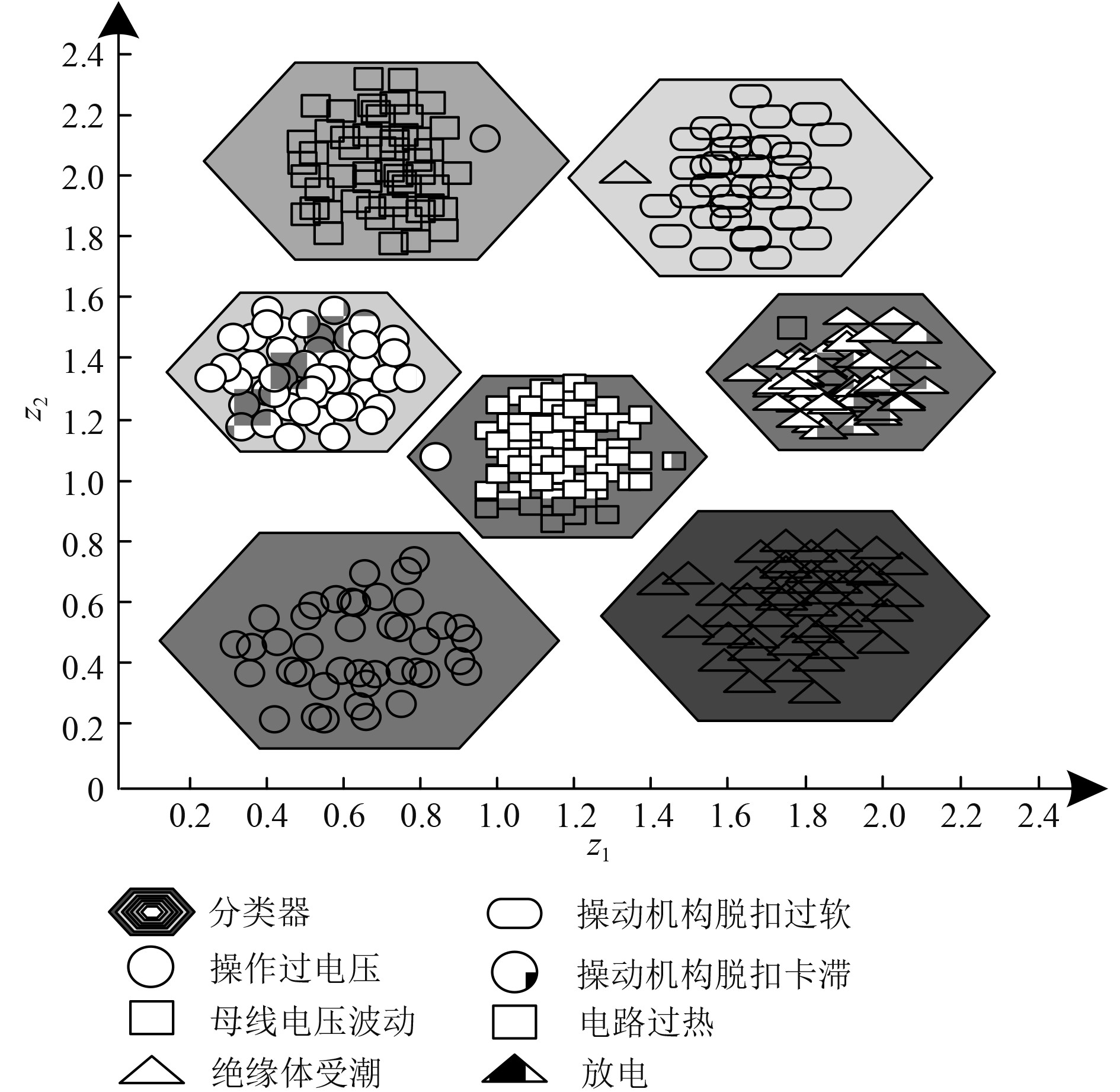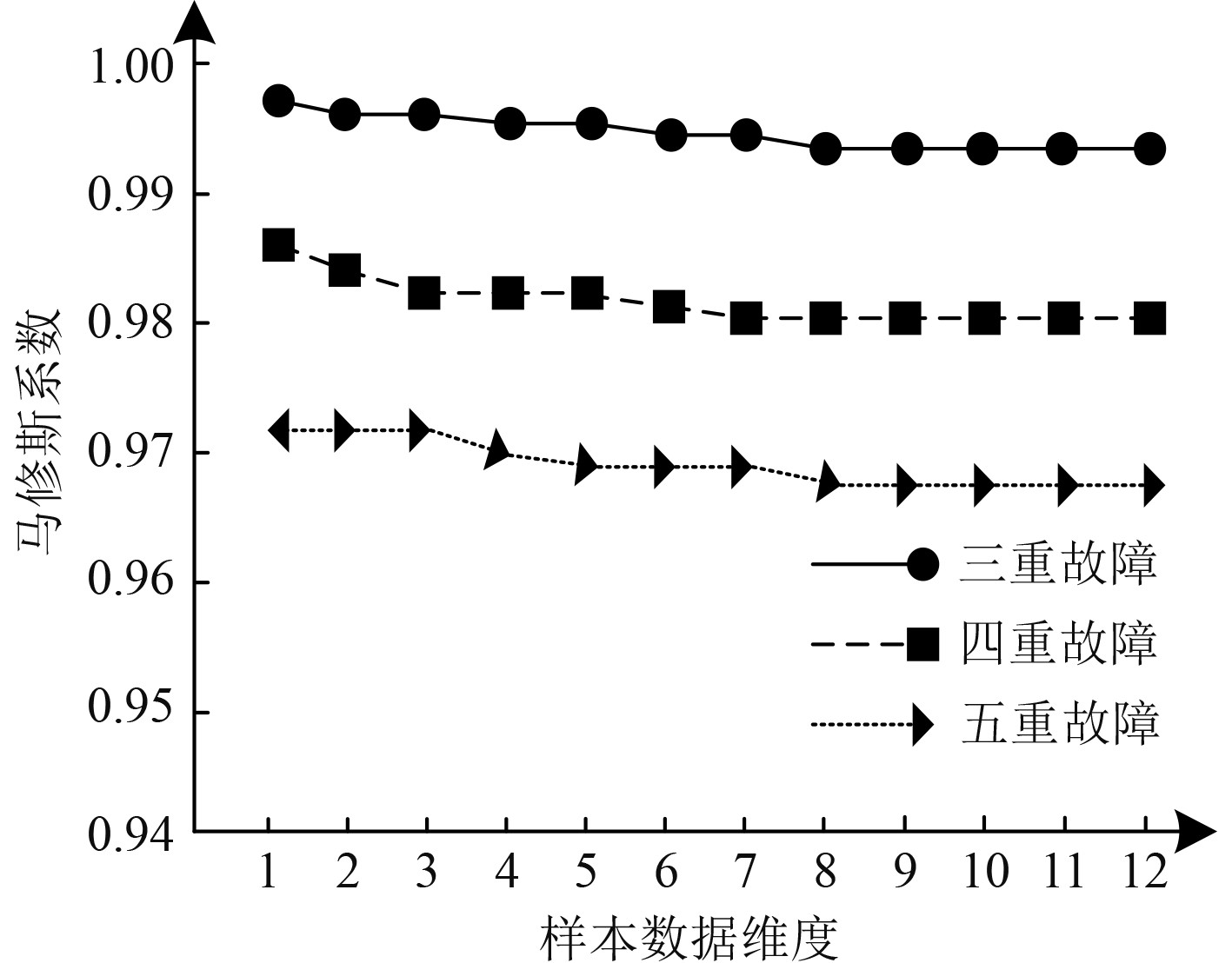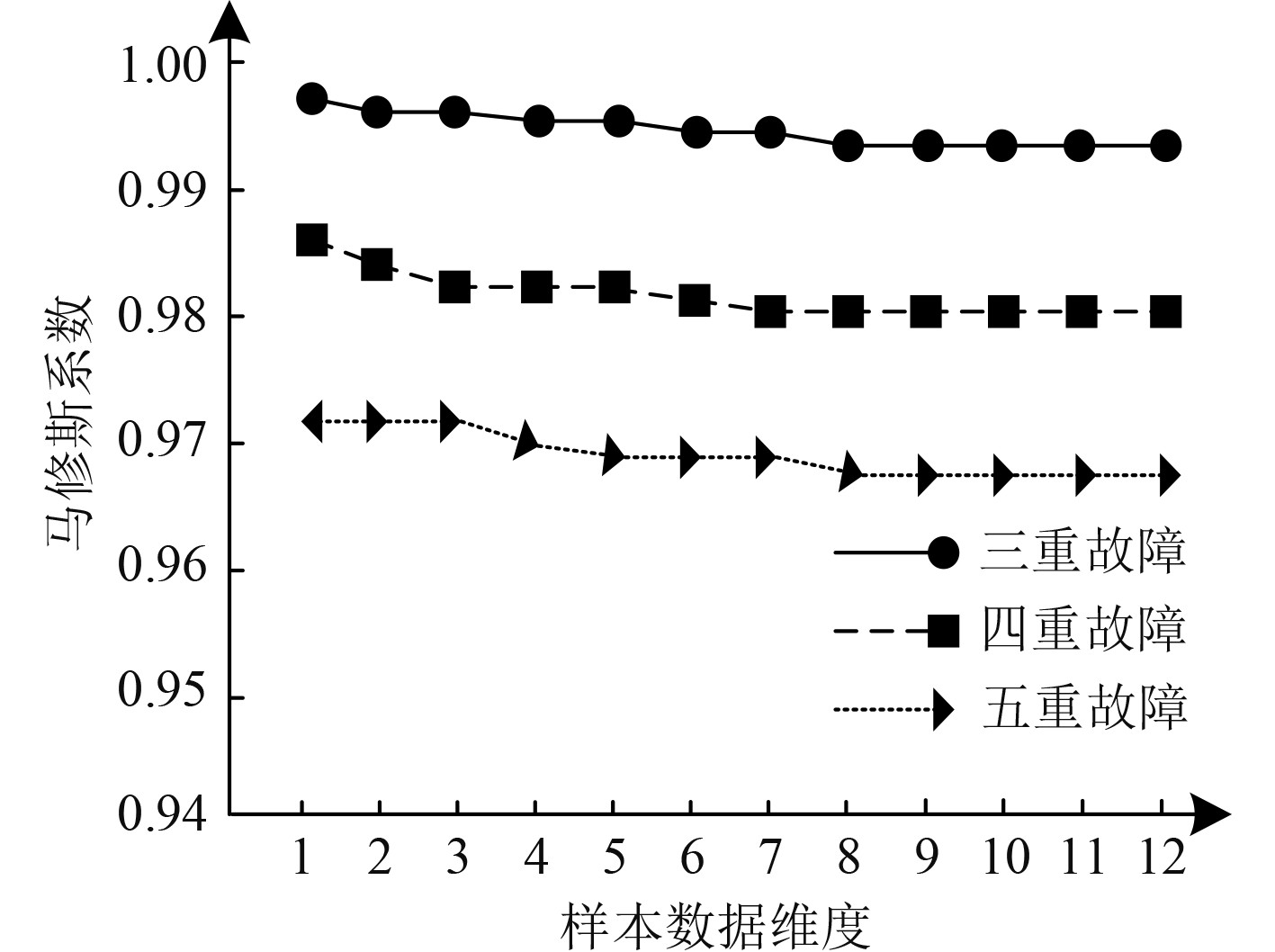﻿ 船舶电气系统绝缘故障自适应诊断技术
 舰船科学技术2022, Vol. 44Issue (14): 123-126    DOI: 10.3404/j.issn.1672-7649.2022.14.026PDF

Adaptive diagnosis technology of insulation fault in marine electrical system
LIN Hang, XIA Peng-fei
Qingdao Branch of China Classification Society, Qingdao 266034, China
Abstract: In order to reasonably reduce the characteristic value of insulation fault and accurately and adaptively diagnose insulation fault, an adaptive diagnosis technology of insulation fault of ship electrical system is proposed. The kernel principal component analysis method is used to reduce the dimension of insulation fault eigenvalues and input them into support vector machine. Based on the principle of marginal maximization, the optimal partition hyperplane of support vector machine is established, and the optimal partition hyperplane is solved by introducing Lagrange function to obtain the adaptive diagnosis results of insulation fault. Particle swarm optimization algorithm is used to optimize the parameters of support vector machine to improve the effect of fault adaptive diagnosis. The experimental results show that this technology can effectively reduce the eigenvalue dimension of insulation fault and accurately and adaptively diagnose various insulation faults. Under different sample dimensions and insulation fault scale, the Matthews coefficient of insulation fault diagnosis of this technology is higher, that is, the accuracy of insulation fault diagnosis is higher.
Key words: ship power system     insulation failure     adaptive     diagnostic technology     the principal components     vector machine
0 引　言

1 船舶电气系统绝缘故障诊断 1.1 核主成分分析的船舶电气系统绝缘故障特征降维

 $E = \frac{{\displaystyle\sum\limits_i {{x_i}x_i^{\rm{T}}} }}{n} = \frac{{X{X^{\rm{T}}}}}{n} 。$ (1)

 $EZ = \sum\limits_a {{\lambda _a}\gamma {z_a}z_a^{\rm{T}}} 。$ (2)

 $\left\{ \begin{gathered} {y_1} = \gamma z_1^T{x_1}，\\ {y_2} = \gamma z_2^T{x_2}，\\ \mathop {}\nolimits_{} \mathop {}\nolimits_{} \vdots \\ {y_k} = \gamma z_k^T{x_k} 。\\ \end{gathered} \right.$ (3)

1.2 基于支持向量机的船舶电气系统绝缘故障诊断

${y_i} \in \left\{ { - 1,1} \right\}$ 情况下，属于二类划分问题，在 ${y_i} \in \left\{ {1,2, \cdots ,k} \right\}$ 情况下，属于k类划分，船舶电气系统绝缘故障自适应诊断属于k类划分问题。在 $f\left( z \right)$ 是线性函数情况下，属于线性分类，反之，属于非线性分类。

 ${y_i} = f'\left( {{z_i}} \right) = {\rm sgn} \varphi \left( {{z_i}} \right)\left( {\left( {w \cdot {z_i}} \right) + b} \right) 。$ (4)

 $\min \left( {\left\| {\varphi \left( z \right) - \varphi \left( {{z_i}} \right)} \right\|:z,\left( {w \cdot z} \right) + b = 0} \right)。$ (5)

 $\mathop {\max }\limits_{w \cdot b} \left( {\min \left( {\left\| {\varphi \left( z \right) - \varphi \left( {{z_i}} \right)} \right\|:z,\left( {w \cdot z} \right) + b = 0} \right)} \right) 。$ (6)

 $\left\{ \begin{gathered} \min \frac{{{{\left\| w \right\|}^2}}}{2}，\\ {y_i}\left( {\left( {w \cdot {z_i}} \right) + b} \right) \geqslant 1 。\\ \end{gathered} \right.$ (7)

2 实验分析图 1 操作过电压时的电流数据 Fig. 1 Current data when operating overvoltage表 1 特征向量降维处理结果 Tab.1 Dimension reduction processing results of feature vectors图 2 电气系统绝缘故障自适应诊断结果 Fig. 2 Adaptive diagnosis results of insulation fault of electrical system图 3 故障可信度测试结果 Fig. 3 Failure reliability test results图 4 绝缘故障自适应诊断精度测试结果 Fig. 4 Test results of insulation fault adaptive diagnosis accuracy

3 结　语

  薛征宇, 郑新潮, 邱翔, 等. 基于支持向量机的船舶感应电机轴承故障在线诊断方法[J]. 船海工程, 2020, 49(5): 1-5+9. DOI:10.3963/j.issn.1671-7953.2020.05.001  徐鹏, 杨海燕, 程宁, 等. 基于优化BP神经网络的船舶动力系统故障诊断[J]. 中国舰船研究, 2021, 16(S1): 106-113. DOI:10.19693/j.issn.1673-3185.02453  王涤, 马爱军, 归宇, 等. 基于P-CNN的局部放电绝缘故障融合诊断[J]. 高电压技术, 2020, 46(8): 2897-2905. DOI:10.13336/j.1003-6520.hve.20190118  吴建波, 王春艳, 洪华军, 等. 基于极限学习机的船舶柴油机故障诊断[J]. 计算机工程与应用, 2019, 55(15): 147-152.  臧旭, 马宏忠, 吴金利, 等. 基于改进集总经验模态近似熵的GIS放电故障诊断[J]. 高压电器, 2020, 56(6): 129-137. DOI:10.13296/j.1001-1609.hva.2020.06.019  张育炜, 石琦, 武恩光. 基于支持向量机GIS局放小波包能量谱故障诊断[J]. 电气传动, 2020, 50(9): 99-105+114.